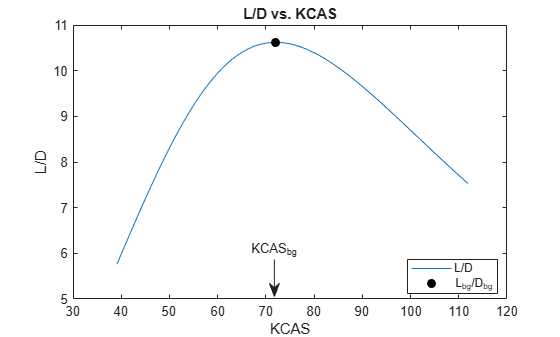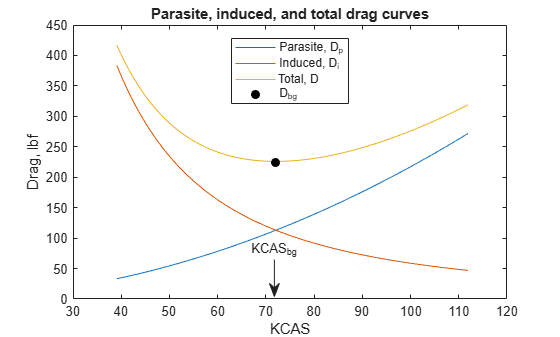# Calculating Best Glide Quantities

This example shows how to perform glide calculations for a Cessna 172 following Example 9.1 in Reference 1 using the Aerospace Toolbox software.

Best glide calculations provide values (velocity and glide angle) that minimize drag and maximize lift-drag ratio (also called the glide ratio).

### Aircraft Specifications

The aircraft parameters are declared as follows.

```W = 2400; % weight, lbf S = 174; % wing reference area, ft^2; A = 7.38; % wing aspect ratio C_D0 = 0.037; % flaps up parasite drag coefficient e = 0.72; % airplane efficiency factor```

### Conditions

Set the current aircraft conditions. The bank angle (phi) is zero for this case.

```h = 4000; % altitude, ft phi = 0; % bank angle, deg```

Convert altitude to meters using `convlength`. The atmospheric calculations in the next step require values in metric units.

`h_m = convlength(h,'ft','m');`

Calculate atmospheric parameters based on altitude using `atmoscoesa`:

`[T, a, P, rho] = atmoscoesa(h_m, 'Warning');`

Convert density from metric to English units using `convdensity`:

`rho = convdensity(rho,'kg/m^3','slug/ft^3');`

### Best Glide Data

Best glide velocity is calculated using the following equation. TAS (true airspeed in feet per second) is the velocity of the aircraft relative to the surrounding air mass.

`$TA{S}_{bg}=\sqrt{\frac{2W}{\rho S}}×\left[\frac{1}{4{{C}_{{D}_{0}}}^{2}+{C}_{{D}_{0}}\pi eA{\mathrm{cos}}^{2}\varphi }{\right]}^{\frac{1}{4}}$`

```TAS_bg = sqrt( (2*W)/(rho*S) )... *( 1./( (4*C_D0.^2) + (C_D0.*pi*e*A*(cos(phi)^2)) )).^(1/4); % TAS, fps```

Convert velocity from fps to kts using `convvel`. KTAS is true airspeed in knots.

`KTAS_bg = convvel(TAS_bg,'ft/s','kts')';`

Convert KTAS to KCAS using `correctairspeed`. KCAS (calibrated airspeed in knots) is the velocity corrected for instrument error and position error. This position error comes from inaccuracies in static pressure measurements at different points in the flight envelope.

`KCAS_bg = correctairspeed(KTAS_bg,a,P,'TAS','CAS')';`

Best glide angle is calculated using:

`$\mathrm{sin}{\gamma }_{bg}=-\sqrt{\frac{4{C}_{{D}_{0}}}{\pi eA{\mathrm{cos}}^{2}\varphi +4{C}_{{D}_{0}}}}$`

This is the angle between the flight path and the ground that provides the highest L/D ratio.

`gamma_bg_rad = asin( -sqrt((4.*C_D0')./(pi*e*A*cos(phi)^2 + 4.*C_D0')) );`

Convert glide angle from radians to degrees using `convang`:

`gamma_bg = convang(gamma_bg_rad,'rad','deg');`

Best glide drag is calculated using:

`${D}_{min}={D}_{bg}=\frac{1}{2}\rho \left(TA{S}_{bg}^{2}\right)S\left(2{C}_{{D}_{0}}\right)=-W\mathrm{sin}{\gamma }_{bg}$`

`D_bg = -W*sin(gamma_bg_rad);`

Best glide lift is calculated using:

`${L}_{bg}={L}_{max}=W\mathrm{cos}{\gamma }_{bg}=\sqrt{{W}^{2}-{D}_{bg}^{2}}$`

`L_bg = W*cos(gamma_bg_rad);`

Calculate dynamic pressure using `dpressure`:

`qbar = dpressure([TAS_bg' zeros(size(TAS_bg,2),2)], rho);`

Calculate drag and lift coefficients using:

`${C}_{{D}_{bg}}=\frac{{D}_{bg}}{\underset{}{\overset{‾}{q}}S}$`

`C_D_bg = D_bg./(qbar*S);`

`${C}_{{L}_{bg}}=\frac{{L}_{bg}}{\underset{}{\overset{‾}{q}}S}$`

`C_L_bg = L_bg./(qbar*S);`

### Summary of Best Glide Values

Here are the best glide values:

`$KCA{S}_{bg}=71.9KCAS$`

`${\gamma }_{bg}=-5.38\mathrm{deg}$`

`${C}_{{D}_{bg}}=0.074$`

`${C}_{{L}_{bg}}=0.7859$`

`${D}_{bg}=224.9lbf$`

`${L}_{bg}=2389.4lbf$`

### Verification

These plots show drag and lift-drag ratio plots for the aircraft as a function of KCAS. The plots are used to verify the best glide calculations.

Set range of airspeeds and convert to KCAS using `convvel` and `correctairspeed`:

```TAS = (70:200)'; % true airspeed, fps KTAS = convvel(TAS,'ft/s','kts')'; % true airspeed, kts KCAS = correctairspeed(KTAS,a,P,'TAS','CAS')'; % corrected airspeed, kts```

Calculate dynamic pressure for new airspeeds using `dpressure`:

`qbar = dpressure([TAS zeros(size(TAS,1),2)], rho);`

Calculate parasite drag using:

`${D}_{p}=\frac{1}{2}\rho S{C}_{{D}_{0}}\left(TA{S}^{2}\right)$`

`Dp = qbar*S.*C_D0;`

Calculate induced drag using:

`${D}_{i}=\frac{2{W}^{2}}{\rho S\pi eA}\frac{1}{\left(TA{S}^{2}\right)}$`

`Di = (2*W^2)/(rho*S*pi*e*A).*(TAS.^-2);`

Calculate total drag using:

`$D={D}_{p}+{D}_{i}$`

`D = Dp + Di;`

Approximate lift as weight (assuming small glide angle and small angle of attack). At this speed, assuming

`${C}_{L}=2\pi \alpha$`

and using

`${C}_{{L}_{bg}}$`

from above, the angle of attack is about 7 degrees. Adding the flight path angle (i.e. best glide angle) from above shows the fuselage pitch (attitude angle theta) to be about 2 degrees.

`L = W;`

Plot L/D versus KCAS

As expected, the maximum L/D occurs at approximately the best glide velocity calculated above.

```h1 = figure; plot(KCAS,L./D); title('L/D vs. KCAS'); xlabel('KCAS'); ylabel('L/D'); hold on plot(KCAS_bg,L_bg/D_bg,'Marker','o','MarkerFaceColor','black',... 'MarkerEdgeColor','black','Color','white'); hold off legend('L/D','L_{bg}/D_{bg}','Location','Best'); annotation('textarrow',[0.49 0.49],[0.23 0.12],'String','KCAS_{bg}');```Plot parasite, induced, and total drag curves

Notice the minimum total drag (i.e. D_bg) occurs at approximately the same best glide velocity calculated above.

```h2 = figure; plot(KCAS,Dp,KCAS,Di,KCAS,D); title('Parasite, induced, and total drag curves'); xlabel('KCAS'); ylabel('Drag, lbf'); hold on plot(KCAS_bg,D_bg,'Marker','o','MarkerFaceColor','black',... 'MarkerEdgeColor','black','Color','white'); hold off legend('Parasite, D_p','Induced, D_i','Total, D','D_{bg}','Location','Best'); annotation('textarrow',[0.49 0.49],[0.23 0.12],'String','KCAS_{bg}');````close(h1,h2);`

### Reference

``` Lowry, J. T., "Performance of Light Aircraft", AIAA(R) Education Series, Washington, DC, 1999. ```